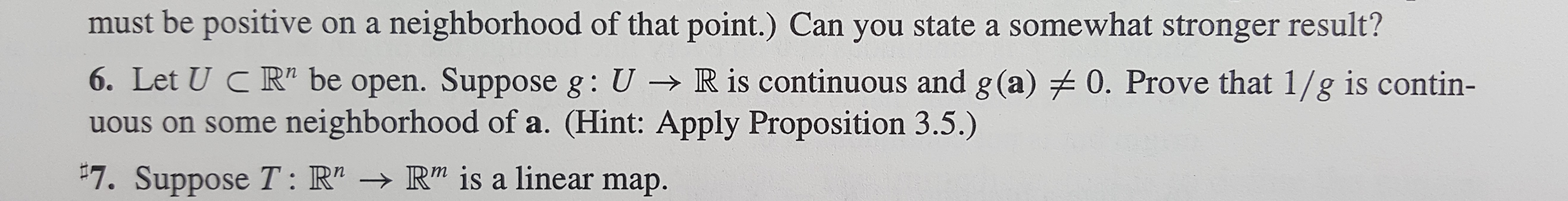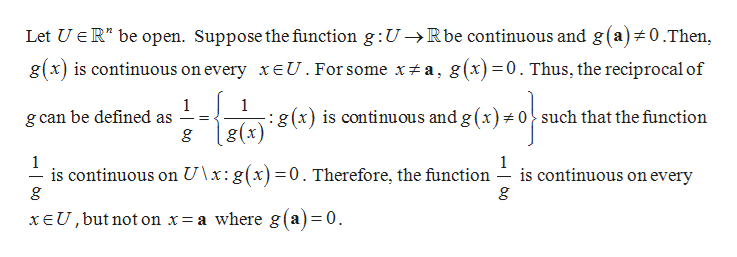# must be positive on a neighborhood of that point.) Can you state a somewhat stronger result?6. Let U C R" be open. Suppose g: U R is continuous and g(a) 0. Prove that 1/g is contin-us on some neighborhood of a. (Hint: Apply Proposition 3.5.)7. Suppose T: R"-R" is a linear map.

Question

I need help for problem 6. Thank you very much!help_outlineImage Transcriptionclosemust be positive on a neighborhood of that point.) Can you state a somewhat stronger result? 6. Let U C R" be open. Suppose g: U R is continuous and g(a) 0. Prove that 1/g is contin- us on some neighborhood of a. (Hint: Apply Proposition 3.5.) 7. Suppose T: R"-R" is a linear map. fullscreen
check_circle

Step 1

To prove:

Step 2

Proof:

...help_outlineImage TranscriptioncloseLet UER" be open. Suppose the function g:U->Rbe continuous and g (a)# 0.Then, g(x) is continuous on every xeU. For some x# a, g(x)=0. Thus, the reciprocal of 1 g can be defined as 1 :g(x) is continuous and g (x)# o^ such that the function g(x) 1 is continuous on U\x: g(x)=0. Therefore, the function g 1 is continuous on every xEU, but not on x= a where g(a)=0 fullscreen

### Want to see the full answer?

See Solution

#### Want to see this answer and more?

Solutions are written by subject experts who are available 24/7. Questions are typically answered within 1 hour.*

See Solution
*Response times may vary by subject and question.
Tagged in

### Math